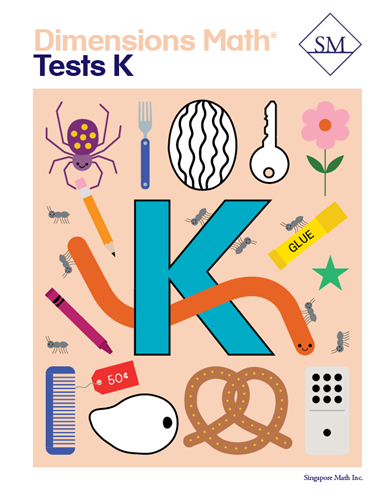# Dimensions Math Tests K

\$ 12.00

Kindergarten A

Test 1 - Chapter 1 Match, Sort, and Classify
Test 2 - Chapter 2 Numbers to 5 (Lessons 1–5)
Test 3 - Chapter 2 Numbers to 5 (Lessons 6–11)
Test 4 - Chapter 3 Numbers to 10 (Lessons 1–6)
Test 5 - Chapter 3 Numbers to 10 (Lessons 7-12)
Test 6 - Chapter 4 Shapes and Solids (Lessons 1–8)
Test 7 - Chapter 4 Shapes and Solids (Lessons 9–11)
Test 8 - Chapter 5 Compare Height, Length, Weight,and Capacity (Lessons 1–4)
Test 9 - Chapter 5 Compare Height, Length, Weight,and Capacity (Lessons 5–9)
Test 10-Chapter 6 Comparing Numbers Within 10

Kindergarten B

Test 11-Chapter 7 Numbers to 20 (Lessons 1–5)
Test 12-Chapter 7 Numbers to 20 (Lessons 6–9)
Test 13-Chapter 8 Number Bonds (Lessons 1–6)
Test 14-Chapter 8 Number Bonds (Lessons 7–11)
Test 15-Chapter 9 Addition (Lessons 1–6)
Test 16-Chapter 9 Addition (Lessons 7–11)
Test 17-Chapter 10 Subtraction (Lessons 1–5)
Test 18-Chapter 10 Subtraction (Lessons 6–9)
Test 19-Chapter 11 Addition and Subtraction
Test 20-Chapter 12 Numbers to 100 (Lesson 1-6)
Test 21-Chapter 12 Numbers to 100 (Lesson 7-10)
Test 22-Chapter 13 Time
Test 23-Chapter 14 Money

Dimensions Math® PreK-5 series features the progression, rigor, and pacing that define Singapore math. Throughout the series, five characters offer students suggestions on how to think about problems. They remind students of strategies they’ve learned and point out important information that encourages them to come up with their own solutions.

Textbook lessons begin with a task that allows students to apply their previous knowledge and learn through discussion. Once students have mastered a concept with the use of concrete and pictorial aids, they are ready to take on more abstract mathematical problem sets. They reach fluency by collecting various strategies along the way and applying them to new problems. Word problems give students a sense of math in real-world contexts.

Workbooks offer independent practice that follows a careful progression of exercise variation. Each textbook lesson includes a corresponding workbook exercise that starts with pictorial representation and progresses to more challenging abstract problems. Workbooks for PreK-2 are perforated.

Teacher’s Guides include lesson plans, mathematical background, games, helpful suggestions, and comprehensive resources for daily lessons. Lessons are laid out clearly and activities are designed for the whole class, small groups, and extension.

Textbooks and Workbooks do not include answer keys. Answers are in Teacher's Guides.
Textbook:
ISBN  9781947226029
pp 152
Workbook:
ISBN  9781947226166
pp 160